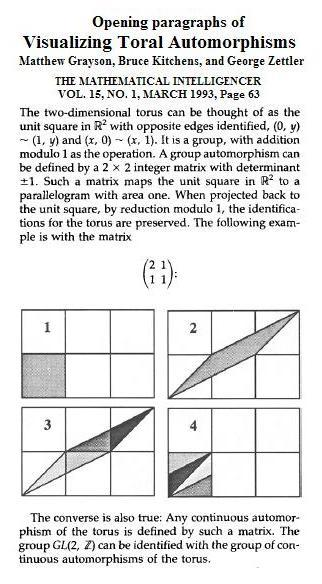Finite Geometry Notes   | Home | Site Map | Author |

### Visualizing GL(2,p)

This web page contains a note from 1985 followed by remarks on some of the groups, and a non-Desarguesian translation plane, implicit in that note. --Steven H. Cullinane, August 28, 2005

December 10, 2008--
For a neater version of the pictures in the 1985 note, see GL(2,3), by Nick Wedd.

August 19, 2009--
For a program by Ed Pegg Jr. at Wolfram.com that extends the example from 1985 shown below to  many, many more group actions on small coordinate arrays, see Group Actions, 1984-2009.

August 19-20, 2009--
The above-mentioned group actions illustrated at Wolfram.com-- GL(2,p) where p keeps increasing-- suggest a natural modification of the 1985 note "Visualizing GL(2,p)"-- Replace p by Z. See "Visualizing Toral Automorphisms," by Matthew Grayson, Bruce Kitchens, and George Zettler, Mathematical Intelligencer, Volume 15, Number 1, March 1993 (illustrated below):
"The group GL(2,Z) can be identified with the group of continuous automorphisms of the torus."
Historically, of course, visualization of GL(2,Z) preceded that of GL(2,p). See, for instance, the Wikipedia article on Arnold's Cat Map and especially its illustration (animated gif, 3.5 mb) of a discrete version of an iterated toral automorphism applied to a picture of a pepper.

Steven H. Cullinane

Visualizing GL(2,p).  Expository Note.  March 26, 1985

"The typical example of a finite group is GL(n,q), the general linear group of n dimensions over the field with q elements." -- J. L. Alperin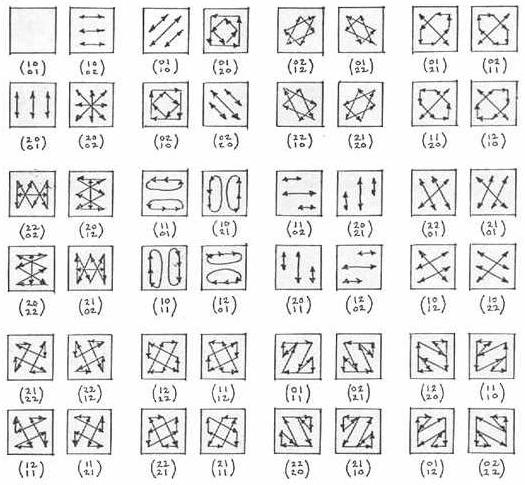The 48 actions of GL(2,3) on a 3x3 coordinate-array A are illustrated above. The matrices shown right-multiply the elements of A, where

 A = (1,1) (1,0) (1,2) (0,1) (0,0) (0,2) (2,1) (2,0) (2,2)

Actions of GL(2,p) on a pxp coordinate-array have the same sorts of symmetries, where p is any odd prime.

Quaternions

It is well known that the quaternion group is a subgroup of GL(2,3), the general linear group on the 2-space over GF(3), the 3-element Galois field.

The figures below illustrate this fact. (Here the "2" of the note above is replaced by its equivalent, modulo 3: "-1.")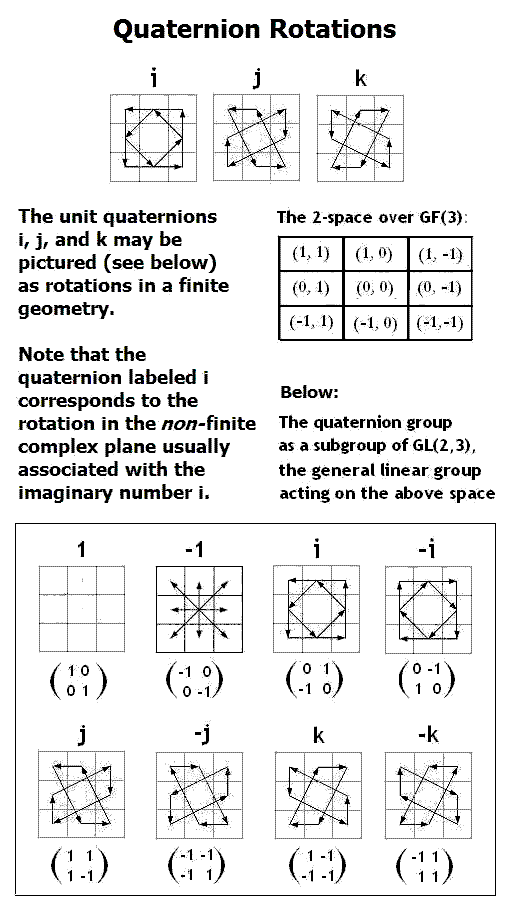The 24-Cell

Visualizing the
binary tetrahedral group
(the 24-cell):Another representation of
the 24-cell
: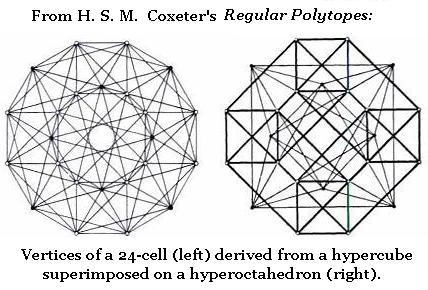From John Baez, "This Week's Finds in Mathematical Physics (Week 198)," September 6, 2003:  Noam Elkies writes to John Baez: Hello again, You write: [...] "I'd like to wrap up with a few small comments about last Week.  There I said a bit about a 24-element group called the 'binary tetrahedral group', a 24-element group called SL(2,Z/3), and the vertices of a regular polytope in 4 dimensions called the '24-cell'.  The most important fact is that these are all the same thing! And I've learned a bit more about this thing from here:" [...] Here's yet another way to see this: the 24-cell is the subgroup of the unit quaternions (a.k.a. SU(2)) consisting of the elements of norm 1 in the Hurwitz quaternions - the ring of quaternions obtained from the Z-span of {1,i,j,k} by plugging up the holes at (1+i+j+k)/2 and its <1,i,j,k> translates. Call this ring A. Then this group maps injectively to A/3A, because for any g,g' in the group |g-g'| is at most 2 so g-g' is not in 3A unless g=g'. But for any odd prime p the (Z/pZ)-algebra A/pA is isomorphic with the algebra of 2*2 matrices with entries in Z/pZ, with the quaternion norm identified with the determinant. So our 24-element group injects into SL2(Z/3Z) - which is barely large enough to accommodate it. So the injection must be an isomorphism. Continuing a bit longer in this vein: this 24-element group then injects into SL2(Z/pZ) for any odd prime p, but this injection is not an isomorphism once p>3. For instance, when p=5 the image has index 5 - which, however, does give us a map from SL2(Z/5Z) to the symmetric group of order 5, using the action of SL2(Z/5Z) by conjugation on the 5 conjugates of the 24-element group. This turns out to be one way to see the isomorphism of PSL2(Z/5Z) with the alternating group A5. Likewise the octahedral and icosahedral groups S4 and A5 can be found in PSL2(Z/7Z) and PSL2(Z/11Z), which gives the permutation representations of those two groups on 7 and 11 letters respectively; and A5 is also an index-6 subgroup of PSL2(F9), which yields the identification of that group with A6. NDE

Translation Plane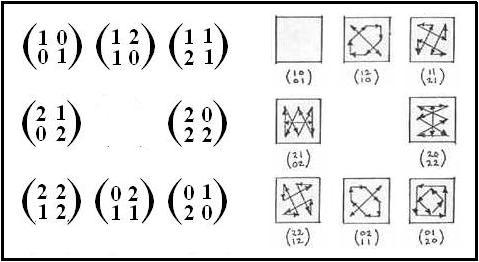Figure A

 From the website of The Translation Plane of Order Nine There are exactly four projective planes of order nine, and one of these planes is a non-Desarguesian translation plane. Theorem. Up to isomorphism, there exists exactly one non-Desarguesian translation plane of order 9. This translation plane is defined by a spreadset in a 2-dimensional vector space over the field GF(3), consisting of the following matrices.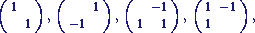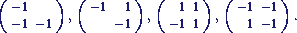As it turns out, the coordinatizing quasifield is a nearfield. Moreover the non-Desarguesian translation plane of order 9 has Lenz-Barlotti type IVa.3.

Two versions of the defining spreadset for this plane are shown in Figure A.  In the left part of Fig. A, the matrices of Dr. Klein are altered by the use of "2" instead of "-1" (since these are the same, modulo 3).  In the right part of Fig. A, the corresponding figures from my 1985 note Visualizing GL(2, p) are shown.

A natural modification of
"Visualizing GL(2,p)"--
"Visualizing GL(2, Z)."